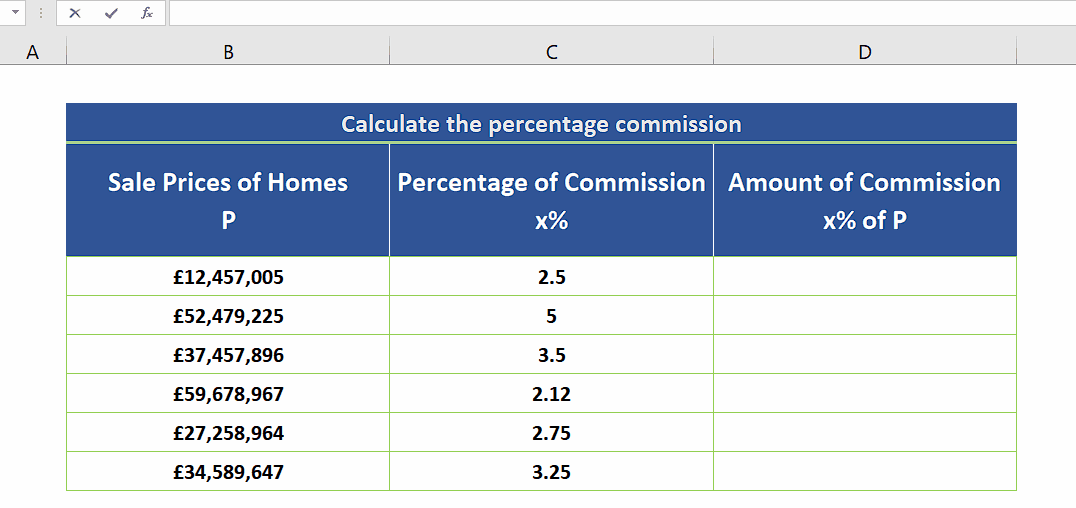# How to calculate the percentage of a number in Excel

Mathematically it is a very easy procedure to calculate the percentage of a number. The formula is very simple which is given below;

Number = N

Percentage to calculate = x

Result = N * (x / 100)

In this tutorial we’ll learn how to perform this simple calculation in Excel. Let’s explore the dataset shown above. It has the data for the sale prices of various homes and we need to calculate the percentage commission for each deal.

We’ll create the formula manually and then implement it to the whole data range. We have the prices in one Column and the percentage commissions in another Column. So, let’s create the formula and calculate the percentage commissions by following the steps mentioned below.Excel has very powerful features for handling text, numeric and alphanumeric data as well. It has many functions not only for computation but also for visualization and data analysis. Sometimes, while handling numeric data we need to calculate the percentage of numbers.

### Step 1 – Create the formula and calculate the percentage commission– We can use the following simple formula for calculating the percentage commissions,
=B4 * C4 / 100
– Double click the fill handle to copy the formula down the whole data range as shown above.